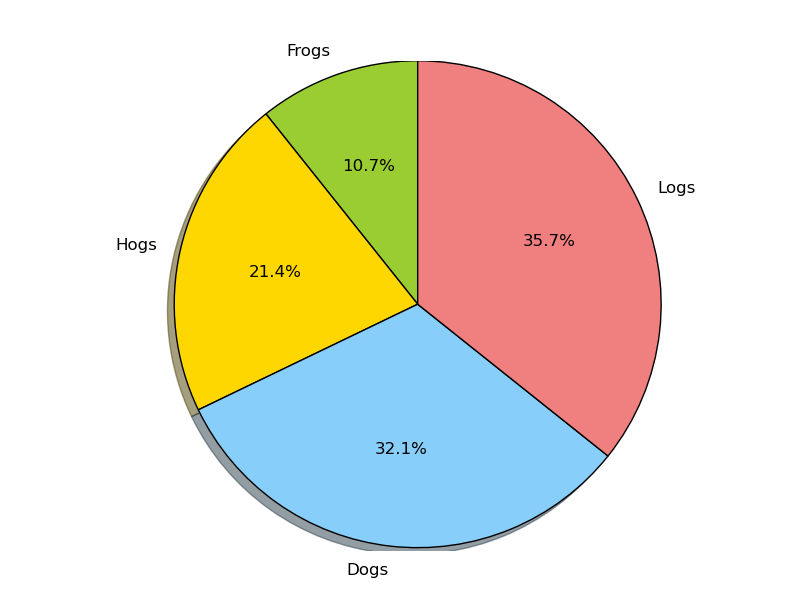## Piechart

Date: March 10th 2016
Last updated: March 10th 2016

This is for future reference how to add exploded pieces to a piechart. The example is available from here http://matplotlib.org/examples/pie_and_polar_charts/pie_demo_features.html.

main.py

``````import matplotlib.pyplot as plt

# The slices will be ordered and plotted counter-clockwise.
labels = 'Frogs', 'Hogs', 'Dogs', 'Logs'
# create a list
sizes = [15, 30, 45, 50]
colors = ['yellowgreen', 'gold', 'lightskyblue', 'lightcoral']

# NOT EXPLODED
plt.pie(sizes, labels=labels,
colors=colors,
autopct='%1.1f%%',  #<==== pie slice percentage accuracy (1 decimal place)
shadow=True,
startangle=90)
# EXPLODED
# explode = (0, 0.1, 0, 0)  # only "explode" the 2nd slice (i.e. 'Hogs')
#plt.pie(sizes, explode=explode, #<==== explode
#               labels=labels,
#               colors=colors,
#               autopct='%1.1f%%',
#               shadow=True,
#               startangle=90)

# Set aspect ratio to be equal so that pie is drawn as a circle.
plt.show()
``````

Output